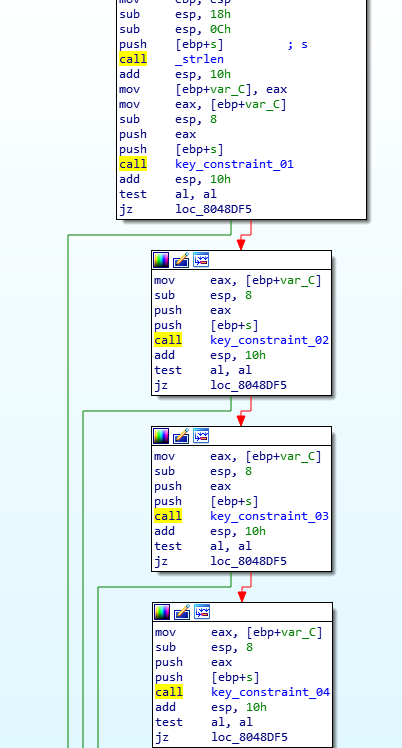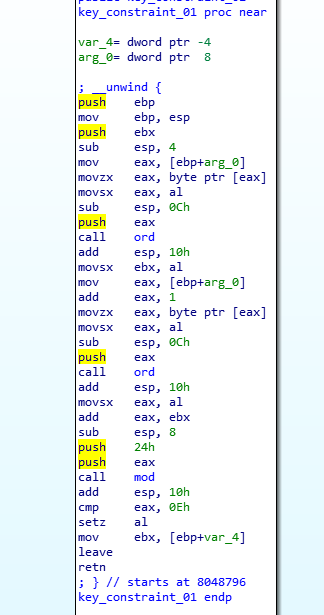18 October 2018

# PicoCTF 2018 - KeyGen 2 WriteUp

by XxcoralloxX

This is a more advanced version of KeyGen 1.

In order to make this writeUp shorter, I will skip parts equal to keyGen 1.

So please, have a look at that before:

https://pwnthemole.github.io/reverse/2018/10/18/PicoCTF2018-KeyGen1.html

Here we have the same “check_valid_key” function which check if the key is 16 chars long and it has only chars in range (0:9 and A:Z)

But the “validate_key” is different.as you can see, there are several checks on the strings (12). If the string respect all the constraint we get the flag.

constraints are quite easy, here is an example:it uses the same ord function of KeyGen 1 and a mod function (that perform a simple mod operation). in this first example, the constraint is

ord(string)+ord(string)%36 == 14


reversing all the functions, here we have all the constraints

1) return ((sol+sol)%36)==14;
2) return ((sol+sol)%36)==24;
3) return ((sol-sol)%36)==6;
4) return ((sol+sol+sol)%36)==4;
5) return ((sol+sol+sol)%36)==13;
6) return ((sol+sol+sol)%36)==22;
7) return ((sol+sol+sol)%36)==31;
8) return ((sol+sol+sol)%36)==7;
9) return ((sol+sol+sol)%36)==20;
10) return ((sol+sol+sol)%36)==12;
11) return ((sol+sol+sol)%36)==27;
12) return ((sol+sol+sol)%36)==23;


Due to the simplicity of constraints, it’s quite easy to use them in a brute-force with enough pruning.

Here is the link of the script I used:

https://github.com/pwnthemole/ctfs/blob/master/PicoCTF2018-KeyGen2/main.cpp

It produces the key in less than 1 second: 0E6IW8BX07K**Q9D

I also tried to implement this with a z3 script.

here is the script:

https://github.com/pwnthemole/ctfs/blob/master/PicoCTF2018-KeyGen2/z3_script.smt2

it produced a different (but still correct) key, but it took 1 night

XxcoralloxX

tags: reverse  keygen  z3  PicoCTF2018